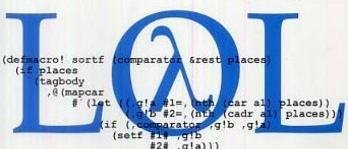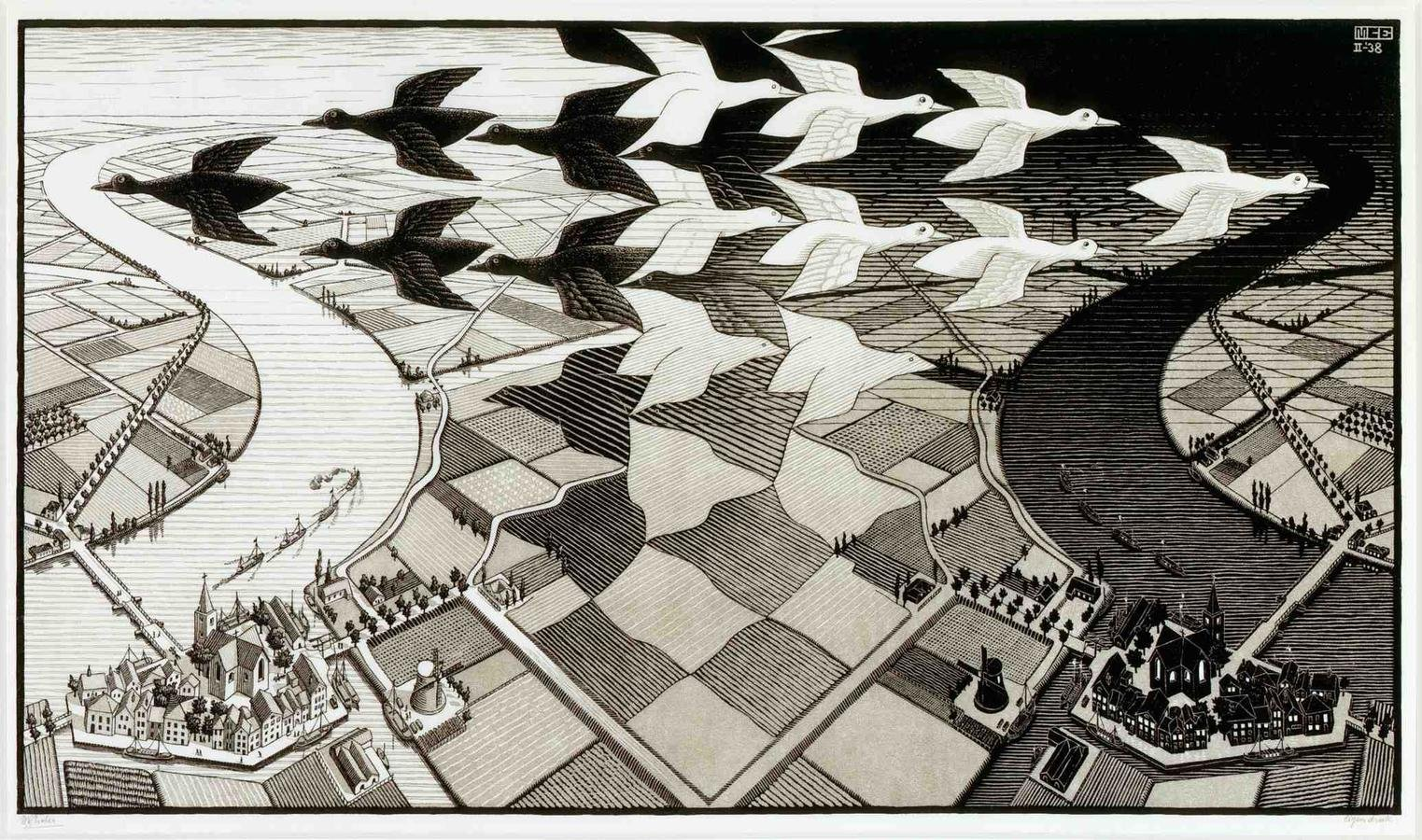# Functional Programming Forever

A novel exploration of symbolic transformations across discrete domains## functional programming ~ * ~ the secret cult of eval & apply ~ * ~Every good wizard knows;

if you have the name of a spirit,

you have power over it.

clojure

js

scala

java

python

λ

## this space reserved for jelly stains

recursionlambdas

symbols

atoms

recursion

λ what is fp ?a style of building the structure and elements of computer programs that treats computation as the evaluation of mathematical functions and avoids state and mutable data

... DUH

λ why ?powerful

expressive

fun

## functional programming

• pure function as the unit of abstraction
• clarity through simplicity
• fancy sounding words

### functional phrases

referential transparencyclosure

lambda

side-effect

higher order function

currying

flatmap

fold

functor

reactive

combinators

metaprogramming

lazy evaluation

macro

bind

parametric types

immutability

# pure functions

map inputs to outputsthat's it...

an impure function

``````# borrowed from garrett smith: http://vimeo.com/97337252

def new_array(element, array=[]):
"""create an array with a starting element"""
array.append(element)
return array

In : new_array(1)
Out: 

In : my_array = new_array(2)
In : my_array.append(3)``````
``````In : my_array
Out: [1, 2, 3]

# python mutable metaprogramming for the wat

In : new_array(1)
Out: [1, 2, 3, 1]``````

a pure function

``````;; thanks clojure

(defn new-array
"create an array with a starting element"
([element]       (new-array element [])
([element array] (conj array element)))

(new-array 1)
; => 

(conj (new-array 2) 3)
; => [2 3]

(new-array 1)
; => ``````

the same inputs always return the same outputsand many more...

imperative

functional

declarative

• patterns for programming
• each is built around different constraints
• ways to think about problems and express solutions# Collections

## out of many, oneCollections in Java

``````
class JavaExample {

List<Integer> incrementedList(List<Integer> numbers) {
for (int i = 0; i < numbers.size(); i++) {
numbers.set(i, numbers.get(i) + 1);
}
return numbers;
}

List<Integer> incrementedList(List<Integer> numbers) {
List<Integer> result = new ArrayList<Integer>();
for (Integer num : numbers) {
}
return result;
}

}``````

(initialization; termination; iteration) vs. (for : each)# Collections in Scala

## mapping over iterables

``````
val inc = { x: Int => x + 1 }

val incrementedList = { xs: Iterable[Int] => xs.map(inc) }
````````````var nums = [ 1, 2, 3, 4, 5, 6];

function even(x) {
return x % 2 == 0;
}

nums.filter(even);

// => [ 2, 4, 6 ]``````

Collections in JavaScript

gang of HOF``````(def up-to-ten (range 10))

up-to-ten
; => (0 1 2 3 4 5 6 7 8 9)

(reduce + up-to-ten)
; => 45

(reductions + up-to-ten)
; => (0 1 3 6 10 15 21 28 36 45)``````

Understanding Reduce

variously called foldcollect, or inject## Some Considerations

• reduce transforms iterables into new values
• map & filter are special cases of reduce
• every iteration is either a reduction or a side-effectThe call is coming from inside the code!

``````function reduce(fn, xs, init) {
var result = init;
xs.each(function(x) {
result = fn(result, x);
});
return result;
}

function map(fn, xs) {
return reduce(function(accumulated, element) {
return conj(accumulated, fn(element));
}, xs, []);
}

function filter(fn, xs) {
return reduce(function(accumulated, element) {
return fn(element) ?
conj(accumulated, element) :
accumulated;
}, xs, []);
}``````Building Blocks

``````function Person(name, age) {
return {
name: name,
age: age
};
}

var brothers = [
new Person("Thomas", 25),
new Person("Eric", 16)
];

map(function(person) {
return person["name"];
}, brothers);``````
``````function get(attribute) {
return function(obj) {
return obj[attribute];
};
}

map(get("age"), brothers);
map(get("name"), brothers);``````

Defining "pluck"

``````pluck("age", brothers);

// => [ 25, 16 ];

function pluck(attribute, xs) {
map(get(attribute), xs);
}``````Inverting filter

``````reject(even, range(10));

// is the same as:

filter(odd, range(10));

// even and odd are complementary functions``````
``````function complement(fn) {
// returns the complement of a given function
return function(x) {
return ! f(x);
}
}

function reject(fn, xs) {
return filter(complement(fn), xs);
}``````

## Functions are abstractions## Functional Problem Solving

• find a smaller problem
• define solutions in terms of pure functions
• repeat## Understanding recursion through functional principles

• define a terminal case
• when am I done?
• define an inductive case
• how can I get closer to a my terminal case?``````def factorial(n, accumulation=1):
if n == 1:
# terminal case
return accumulation
else:
# inductive case
return factorial(n-1, accumulation*n)``````
``````def fibonacci(n):
if n < 2:
# terminal case
return n
else:
# inductive case
return fibonacci(n-1) + fibonacci(n-2)``````

Simple examples...

``````def whoops(n):
if n == 0:
# terminal case
return "yay!"
# these cases take us further away!
elif n > 0:
return whoops(n + 1)
else:
return whoops(n - 1)``````

## FP in the wild

Parallel Processing• MapReduce
• Samza
• Spark
• Storm

Divide and conquer!

# Spark Example

``````# count all the words in hdfs

sentences = spark.textFile("hdfs://...")
word_counts = sentences.flatMap(lambda line: line.split(" "))
.map(lambda word: (word, 1))
.reduceByKey(lambda a, b: a + b)``````

## FP in the wild

Functional UIs

• React
• Elm
• Om
• PureScript
• Bacon.js## react.js example

``````// from: https://gist.github.com/jordwalke/6350319

var PeopleList = React.createClass({

render: function() {
var friends = this.props.friends;
var followers = this.props.followers;
return div({className: 'list'},

// data pipeline to transform a collection into dom nodes
friends.concat(followers)
.filter(function(person) {return person.isFavorite;})
.sort(function(one, two) {return one.followCount - two.followCount;})
.slice(0, 10)
.map(function(person) {
return div({className: person.isVerified ? 'star' : 'gray'}, person.name);
})

);
}

});
``````

functional, immutable dom

## Tip of the iceberglazy sequences

generics

thinking with types

Has functional programming gone too far?By Thomas Omans

• 3,021